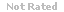Loading... Please wait...

## A concave mirror with a radius of 30 cm creates a real image 40 cm from the mirror. What is the object distance?

Price:
\$7.99

### Product Description

1. A concave mirror with a radius of 30 cm creates a real image 40 cm from the mirror. What is the object distance?
2.  Estimate the average power output of the Sun, given that about 1350 W/m2 reaches the upper atmosphere of the Earth. The distance from the Sun to the Earth is 1.5 × 1011 m.
3.  Which of the equations here is valid for the circuit shown?
4.  Starting from rest, a proton falls through a potential difference of 2700 V. What speed does it acquire?
5.  See the figure. The net force on the 1 nC charge is zero. What is q?
6.  If a current is flowing with a value of 5.9 A, how much electron charge passes any single point in 25 seconds?
7.  A beam of light traveling in air is incident on a transparent plastic at an angle of incidence of 50o. The angle of refraction is 35o. What is the index of refraction of the plastic?
8. If the force between two charges increases by a factor of 16 because the charges are moved closer together, how much closer are they moved?
9.  A long, straight wire carries a current of 2.5 A. Find the magnitude of the magnetic field 25 cm from the wire.
10.  A flux of 4.0 × 10-5 Wb is maintained through a coil for 0.50 s. What emf is induced in this coil by this flux?

### Products by Category

• \$7.99